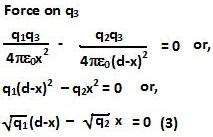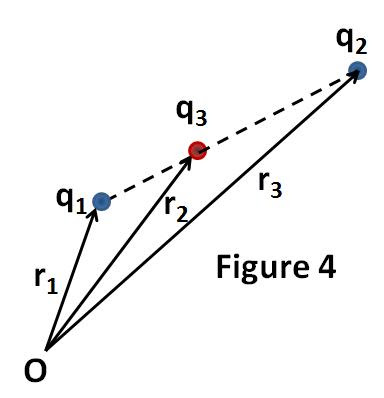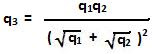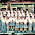## Monday, January 18, 2010

### Irodov Problem 3.4The first thing in this problem is to realize that the third charge q3 has to be collinear with charges q1 and q2. Figure 1 shows a situation when the charges are not collinear. In Figure 1, Fij, represents the force vector acting on charge qi due to qj. In order for the force at q1 to be 0, the vectors F13 and F12 must be equal and opposite. Unless, q3 is in line with q1 and q2, this can never happen. The same argument can be applied to any of the charges.Figure 2 shows the only configuration in which forces can be zero at all three charge locations. Namely, all three charges are collinear and charge q3 is in between charges q1 and q2.

Now let us suppose that q3 is located at a distance x from q1 and that the distance between charges q1 and q2 is d. This is shown in Figure 3.Since the forces acting on the three charges must be zero and q3 is a negative charge we have,It is immediately evident that (3) is redundant since it can be derived from (1) and (2). From (1) and (2) we obtain,In other words, the position of charge q3 divides the line joining charges q1 and q2 into segments of ratio. From elementary coordinate geometry or vectors we know that then the position of charge q3 can be written as,From (4) and (1) we also obtain the value of change q3 as,1.Wrong answer. Please verify with the answer given in the book.

2.Second last step is wrong, the formula used should be

r3 = [root(q1) r2 + root(q2) r1] / root(q1) + root(q2),

Remember it is
x = [ m1x2 + m2x1] / m1 + m2
not
x = [m1x1 + m2x2 ] / m1 + m2